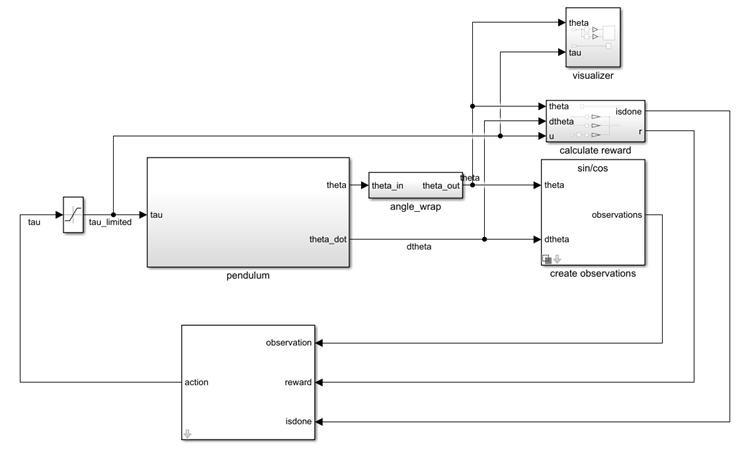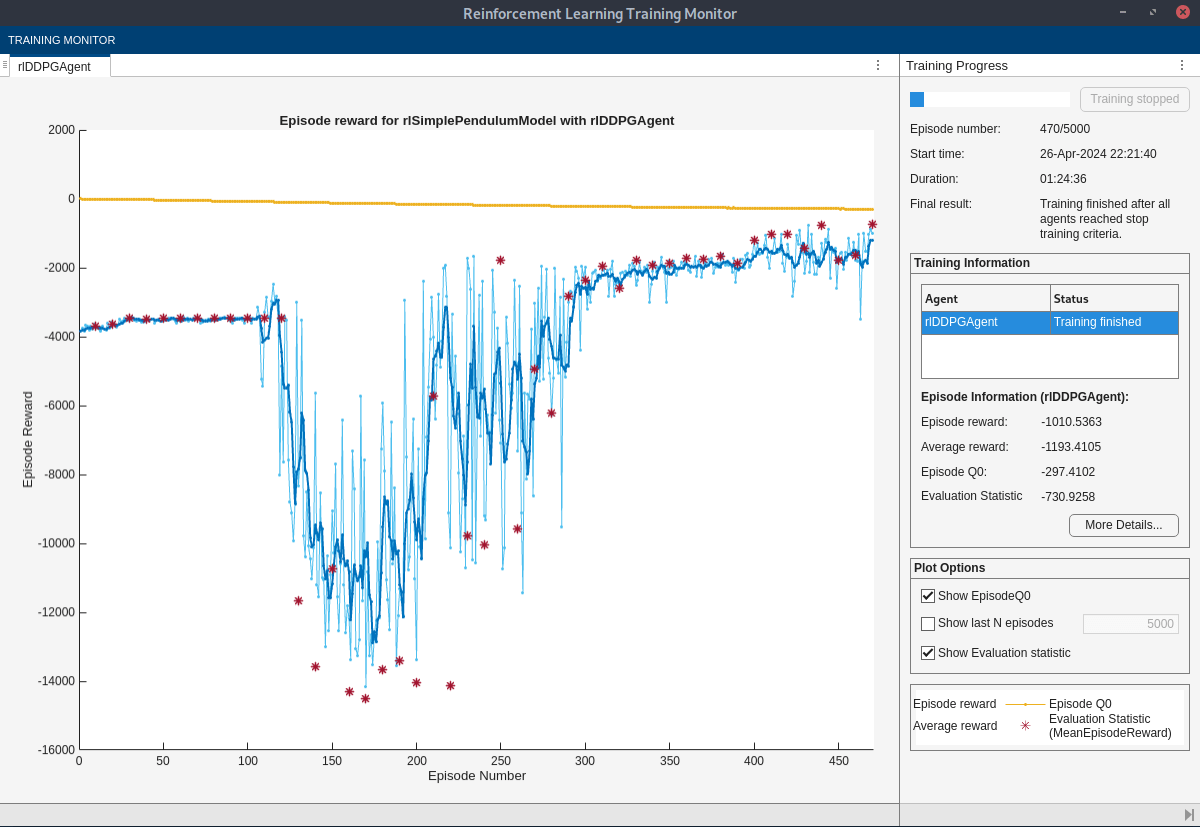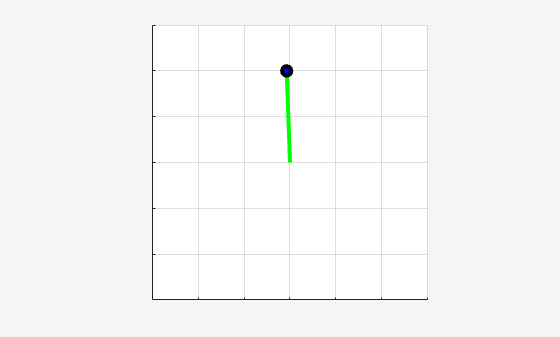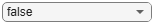# Train DDPG Agent to Swing Up and Balance Pendulum

This example shows how to train a deep deterministic policy gradient (DDPG) agent to swing up and balance a pendulum modeled in Simulink®.

For more information on DDPG agents, see Deep Deterministic Policy Gradient Agents. For an example that trains a DDPG agent in MATLAB®, see Train DDPG Agent to Control Double Integrator System.

### Pendulum Swing-Up Model

The reinforcement learning environment for this example is a simple frictionless pendulum that initially hangs in a downward position. The training goal is to make the pendulum stand upright without falling over using minimal control effort.

Open the model.

```mdl = 'rlSimplePendulumModel'; open_system(mdl)```For this model:

• The upward balanced pendulum position is `0` radians, and the downward hanging position is `pi` radians.

• The torque action signal from the agent to the environment is from –2 to 2 N·m.

• The observations from the environment are the sine of the pendulum angle, the cosine of the pendulum angle, and the pendulum angle derivative.

• The reward ${\mathit{r}}_{\mathit{t}}$, provided at every time step, is

`${\mathit{r}}_{\mathit{t}}=-\left({{\theta }_{\mathit{t}}}^{2}+0.1{\stackrel{˙}{{\theta }_{\mathit{t}}}}^{2}+0.001{\mathit{u}}_{\mathit{t}-1}^{2}\right)$`

Here:

• ${\theta }_{\mathit{t}}$ is the angle of displacement from the upright position.

• $\stackrel{˙}{{\theta }_{\mathit{t}}}$ is the derivative of the displacement angle.

• ${\mathit{u}}_{\mathit{t}-1}$ is the control effort from the previous time step.

### Create Environment Interface

Create a predefined environment interface for the pendulum.

`env = rlPredefinedEnv('SimplePendulumModel-Continuous')`
```env = SimulinkEnvWithAgent with properties: Model : rlSimplePendulumModel AgentBlock : rlSimplePendulumModel/RL Agent ResetFcn : [] UseFastRestart : on ```

The interface has a continuous action space where the agent can apply torque values between –2 to 2 N·m to the pendulum.

Set the observations of the environment to be the sine of the pendulum angle, the cosine of the pendulum angle, and the pendulum angle derivative.

```numObs = 3; set_param('rlSimplePendulumModel/create observations','ThetaObservationHandling','sincos');```

To define the initial condition of the pendulum as hanging downward, specify an environment reset function using an anonymous function handle. This reset function sets the model workspace variable `theta0` to `pi`.

`env.ResetFcn = @(in)setVariable(in,'theta0',pi,'Workspace',mdl);`

Specify the simulation time `Tf` and the agent sample time `Ts` in seconds.

```Ts = 0.05; Tf = 20;```

Fix the random generator seed for reproducibility.

`rng(0)`

### Create DDPG Agent

A DDPG agent approximates the long-term reward, given observations and actions, using a critic value function representation. To create the critic, first create a deep neural network with two inputs (the state and action) and one output. For more information on creating a deep neural network value function representation, see Create Policies and Value Functions.

```statePath = [ featureInputLayer(numObs,'Normalization','none','Name','observation') fullyConnectedLayer(400,'Name','CriticStateFC1') reluLayer('Name', 'CriticRelu1') fullyConnectedLayer(300,'Name','CriticStateFC2')]; actionPath = [ featureInputLayer(1,'Normalization','none','Name','action') fullyConnectedLayer(300,'Name','CriticActionFC1','BiasLearnRateFactor',0)]; commonPath = [ additionLayer(2,'Name','add') reluLayer('Name','CriticCommonRelu') fullyConnectedLayer(1,'Name','CriticOutput')]; criticNetwork = layerGraph(); criticNetwork = addLayers(criticNetwork,statePath); criticNetwork = addLayers(criticNetwork,actionPath); criticNetwork = addLayers(criticNetwork,commonPath); criticNetwork = connectLayers(criticNetwork,'CriticStateFC2','add/in1'); criticNetwork = connectLayers(criticNetwork,'CriticActionFC1','add/in2'); criticNetwork = dlnetwork(criticNetwork);```

View the critic network configuration.

```figure plot(layerGraph(criticNetwork))```Specify options for the critic representation using `rlOptimizerOptions`.

`criticOpts = rlOptimizerOptions('LearnRate',1e-03,'GradientThreshold',1);`

Create the critic representation using the specified deep neural network and options. You must also specify the action and observation info for the critic, which you obtain from the environment interface. For more information, see `rlQValueRepresentation`.

```obsInfo = getObservationInfo(env); actInfo = getActionInfo(env); critic = rlQValueFunction(criticNetwork,obsInfo,actInfo,'ObservationInputNames','observation','ActionInputNames','action');```

A DDPG agent decides which action to take given observations using an actor representation. To create the actor, first create a deep neural network with one input, the observation, and one output, the action.

Construct the actor in a manner similar to the critic. For more information, see `rlDeterministicActorRepresentation`.

```actorNetwork = [ featureInputLayer(numObs,'Normalization','none','Name','observation') fullyConnectedLayer(400,'Name','ActorFC1') reluLayer('Name','ActorRelu1') fullyConnectedLayer(300,'Name','ActorFC2') reluLayer('Name','ActorRelu2') fullyConnectedLayer(1,'Name','ActorFC3') tanhLayer('Name','ActorTanh') scalingLayer('Name','ActorScaling','Scale',max(actInfo.UpperLimit))]; actorNetwork = dlnetwork(actorNetwork); actorOpts = rlOptimizerOptions('LearnRate',1e-04,'GradientThreshold',1); actor = rlContinuousDeterministicActor(actorNetwork,obsInfo,actInfo);```

To create the DDPG agent, first specify the DDPG agent options using `rlDDPGAgentOptions`.

```agentOpts = rlDDPGAgentOptions(... 'SampleTime',Ts,... 'CriticOptimizerOptions',criticOpts,... 'ActorOptimizerOptions',actorOpts,... 'ExperienceBufferLength',1e6,... 'DiscountFactor',0.99,... 'MiniBatchSize',128); agentOpts.NoiseOptions.Variance = 0.6; agentOpts.NoiseOptions.VarianceDecayRate = 1e-5;```

Then create the DDPG agent using the specified actor representation, critic representation, and agent options. For more information, see `rlDDPGAgent`.

`agent = rlDDPGAgent(actor,critic,agentOpts);`

### Train Agent

To train the agent, first specify the training options. For this example, use the following options.

• Run training for at most 50000 episodes, with each episode lasting at most `ceil(Tf/Ts)` time steps.

• Display the training progress in the Episode Manager dialog box (set the `Plots` option) and disable the command line display (set the `Verbose` option to `false`).

• Stop training when the agent receives an average cumulative reward greater than –740 over five consecutive episodes. At this point, the agent can quickly balance the pendulum in the upright position using minimal control effort.

• Save a copy of the agent for each episode where the cumulative reward is greater than –740.

For more information, see `rlTrainingOptions`.

```maxepisodes = 5000; maxsteps = ceil(Tf/Ts); trainOpts = rlTrainingOptions(... 'MaxEpisodes',maxepisodes,... 'MaxStepsPerEpisode',maxsteps,... 'ScoreAveragingWindowLength',5,... 'Verbose',false,... 'Plots','training-progress',... 'StopTrainingCriteria','AverageReward',... 'StopTrainingValue',-740,... 'SaveAgentCriteria','EpisodeReward',... 'SaveAgentValue',-740);```

Train the agent using the `train` function. Training this agent is a computationally intensive process that takes several hours to complete. To save time while running this example, load a pretrained agent by setting `doTraining` to `false`. To train the agent yourself, set `doTraining` to `true`.

```doTraining = false; if doTraining % Train the agent. trainingStats = train(agent,env,trainOpts); else % Load the pretrained agent for the example. load('SimulinkPendulumDDPG.mat','agent') end```### Simulate DDPG Agent

To validate the performance of the trained agent, simulate it within the pendulum environment. For more information on agent simulation, see `rlSimulationOptions` and `sim`.

```simOptions = rlSimulationOptions('MaxSteps',500); experience = sim(env,agent,simOptions);```